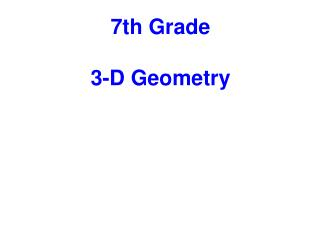DownloadDownload PresentationTélécharger la présentation- - - - - - - - - - - - - - - - - - - - - - - - - - - E N D - - - - - - - - - - - - - - - - - - - - - - - - - - -
##### Presentation Transcript

2. Table of Contents Click on the topic to go to that section 3-Dimensional Solids Cross Sections of 3-Dimensional Figures Volume ·Prisms and Cylinders ·Pyramids, Cones & Spheres Surface Area ·Prisms ·Pyramids ·Cylinders ·Spheres More Practice/ Review Common Core: 7.G.3, 7.G.6, 7.EE.3

4. The following link will take you to a site with interactive 3-D figures and nets.

5. What are three-dimensional figures? • A three-dimensional figure encloses a part of space. • In other words it can hold something (water, air, etc.)

6. Vertex Edge Faces Features of a 3-D figure • Face- the flat surface of a figure • Edge- formed by two faces of a 3-D figure sharing a side • Vertex (plural:vertices)- the point at which three or more edges meet

7. Polyhedron A 3-D figure whose faces are all polygons Sort the figures into the appropriate side. Polyhedron Not Polyhedron

8. 3-Dimensional Solids Categories & Characteristics of 3-D Solids: • Prisms • Have 2 congruent, polygon bases which are parallel • to one another • 2. Sides are rectangular (parallelograms) • 3. Named by the shape of their base click to reveal Pyramids 1. Have 1 polygon base with a vertex opposite it 2. Sides are triangular 3. Named by the shape of their base click to reveal

9. 3-Dimensional Solids Categories & Characteristics of 3-D Solids: • Cylinders • Have 2 congruent, circular bases which are parallel • to one another • 2. Sides are curved click to reveal Cones 1. Have 1 circular bases with a vertex opposite it 2. Sides are curved click to reveal

10. 3-Dimensional Solids Vocabulary Words for 3-D Solids: Polyhedron A 3-D figure whose faces are all polygons (Prisms & Pyramids) Face Flat surface of a Polyhedron Edge Line segment formed where 2 faces meet Vertex (Vertices) Point where 3 or more faces/edges meet

11. 1 Name the figure. A Rectangular Prism Triangular Pyramid B C Hexagonal Prism D Rectangular Pyramid E Cylinder F Cone

12. 2 Name the figure A Rectangular Pyramid B Triangular Prism C Octagonal Prism D Circular Pyramid E Cylinder F Cone

13. 3 Name the figure A Rectangular Pyramid B Triangular Pyramid C Triangular Prism D Hexagonal Pyramid E Cylinder F Cone

14. 4 Name the figure A Rectangular Prism B Triangular Prism C Square Prism D Rectangular Pyramid E Cylinder F Cone

15. 5 Name the figure A Rectangular Prism B Triangular Pyramid C Circular Prism D Circular Pyramid E Cylinder F Cone

16. For each figure, find the number of faces, vertices and edges. Can you figure out a relationship between the number of faces, vertices and edges of 3-Dimensional Figures?

17. Euler's Formula F + V - 2 = E The number of edges is 2 less than the sum of the faces and vertices. click to reveal

18. 6 How many faces does a pentagonal prism have?

19. 7 How many edges does a rectangular pyramid have?

20. 8 How many vertices does a triangular prism have?

22. 3-Dimensional figures can be cut by planes. When you cut a 3-D figure by a plane, the result is a 2-D figure. The cross-sections of 3-D figures are 2 dimensional figures you are familiar with. Look at the example on the next page to help your understanding.

23. A horizontal cross-section of a cone is a circle. Can you describe a vertical cross-section of a cone?

24. A vertical cross-section of a cone is a triangle.

25. A water tower is built in the shape of a cylinder. How does the horizontal cross-section compare to the vertical cross-section?

26. The horizontal cross-section is a circle. The vertical cross-section is a rectangle

27. 9 Which figure has the same horizontal and vertical cross-sections? A C B D

28. 10 Which figure does not have a triangle as one of its cross-sections? A C B D

29. 11 Which is the vertical cross-section of the figure shown? A Triangle B Circle C Square D Trapezoid

30. 12 Which is the horizontal cross-section of the figure shown? A Triangle B Circle C Square D Trapezoid

31. 13 Which is the vertical cross-section of the figure shown? A Triangle B Circle C Square D Trapezoid